# Simple Ramp motion problem, but going full vector mode

• babaliaris
In summary, the conversation discusses solving for the distance a ball will travel until it stops on a frictionless ramp given its mass, initial velocity, air drag, and Earth's acceleration. The solution involves using the ##\Sigma F=ma## equation and simplifying the problem to 1D. The mistake made in the solution involves using vector calculations instead of considering the normal force as a minimum magnitude force preventing interpenetration. The resulting time for the ball to stop may be incorrect due to a possible error in the math calculations. f

#### babaliaris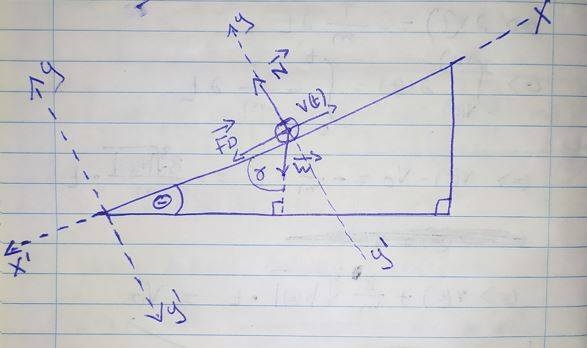Known:
1) The mass of the ball is ##m## (constant ##\frac{dm}{dt} = 0##)
2) ##v(0) = v_{0}##
3) Air drag force magnitude ##| \vec F_{D} | = B \cdot | \vec v(t) |## (##B \in R##)
4) The ramp is frictionless.
5) The magnitude of Earth's acceleration = ##g##

I'm not sure if θ is known or not, and I can't show you the exercise since it is written in Greek.

Looking for the distance that the ball will travel until it stops:
Question: ##s(t)_{max} = ?## @ ##v(t)=0##

My Solution (What is wrong with it?):
##θ + γ + 90 = 180 <=> γ = 90 - θ##
##arg( \vec w ) = 180 + γ = 180 + 90 - θ = 270 - θ##
##\vec F_{D} = -B | \vec v(t)| \vec i##
##\vec w = mg cos(270-θ) \vec i + mg sin(270-θ) \vec j##
##\vec N = mg cos(90) \vec i + mg sin(90) \vec j = mg\vec j##

##Σ \vec F = m \vec a <=> \vec F_{D} + \vec N + \vec W = m \frac{d \vec v(t)}{dt}##
$$<=> -B | \vec v(t) | \vec i + mg \vec j + mg cos(270-θ) \vec i + mg sin(270-θ) \vec j = m \frac{d | \vec v(t) | }{dt} \vec i$$
$$<=> [-B | \vec v(t) | + mg cos(270-θ)] \vec i + [mg + mg sin(270-θ)] \vec j = m \frac{d | \vec v(t) | }{dt} \vec i$$

##-B | \vec v(t) | + mg cos(270-θ) = m \frac{d | \vec v(t) | }{dt}##
##mg + mg sin(270-θ) = 0##

##mg + mg sin(270-θ) = 0 => sin(270-θ) = -1##
##<=> 270-θ = sin^{-1}(-1) = -90 <=> θ = 270+90 = 360##

I don't think ##θ = 360## makes sense so I stopped here... This means that the ramp is not
a ramp at all ##θ = 360 = 0##.

Where is my mistake? Is it in my Physics understanding or a math mistake?

Thank you!

Last edited:
Where is my mistake?
Your first mistake is in using such a complicated approach.
Since there is no friction, we do not care about the normal direction. Consequently, vectors are overkill; it is a 1D problem. Just write the ##\Sigma F=ma## equation for parallel to the plane.

Wrt an actual error:
##\vec N = mg cos(90) \vec i + mg sin(90) \vec j = mg\vec j##
How do you get that?

•babaliaris
I just thought it has the same magnitude as the weight force but different angle. So I thought from the diagram that the angle is 90 and just wrote that.

I know it's a bit overkill. I know how to solve it by analysing weight in two vectors and then going only 1D (what we did in high school) but I really want to understand how to do it with pure vectors.

So how do I figure out the force N as a complete vector?

So how do I figure out the force N as a complete vector?
It helps to understand what a normal force between bodies is: it is the minimum magnitude force that prevents interpenetration. Necessarily, that makes its direction normal to the tangent plane (if there is one; see https://www.physicsforums.com/insights/frequently-made-errors-mechanics-friction/).
Since the bodies here are taken to be incompressible, and the ramp as fixed, that means there is no acceleration normal to the plane. To express that in vectors, you can write, say, ##\vec N.\hat i=0, \vec a.\hat j=0##.

But really, why bother? Use vectors when they're useful.

•babaliaris
I actually figured it out but I believe I still made a mistake somewhere (Probably math-related) because the time that I got, does not seem to be in seconds...

I attached a pdf file since the solution is quite big and I can't write it in latex.

I figured out the following time @ v(t) = 0 (ball stops moving upwards):
##t = - \frac{m}{B} \cdot ln[\frac{m \cdot g \cdot sin(θ)}{m \cdot g \cdot sin(θ)+B \cdot V_{0}}]##
I'm not sure if this is in seconds. I don't even know what units B has.

The enumerator ##mg## is in Newtons. The denominator is also in Newtons ##B\cdot V_{0}## I'm guessing this by the drag formula. So B is in ##\frac{kg}{s}##? So ##\frac{kg}{s} \cdot \frac{m}{s} = \frac{kg \cdot m}{s^{2}} = Newtons##?
This means ##ln[\frac{m \cdot g \cdot sin(θ)}{m \cdot g \cdot sin(θ)+B \cdot V_{0}}]## is just a plain number since ##\frac{N}{N}=1## and it actually must result in a negative number.
So ##\frac{m}{B}## , ##\frac{kg}{\frac{kg}{s}}=s##

If B is indead ##\frac{kg}{s}##, this seems correct.

Teachers/Professors always said that I overcomplicate things. That's how my mind works...

#### Attachments

• ramp solu.pdf
459.8 KB · Views: 45
Last edited:
I actually figured it out but I believe I still made a mistake somewhere (Probably math-related) because the time that I got, does not seem to be in seconds...

I attached a pdf file since the solution is quite big and I can't write it in latex.

I figured out the following time @ v(t) = 0 (ball stops moving upwards):
##t = - \frac{m}{B} \cdot ln[\frac{m \cdot g \cdot sin(θ)}{m \cdot g \cdot sin(θ)+B \cdot V_{0}}]##
I'm not sure if this is in seconds. I don't even know what units B has.

The enumerator ##mg## is in Newtons. The denominator is also in Newtons ##B\cdot V_{0}## I'm guessing this by the drag formula. So B is in ##\frac{kg}{s}##? So ##\frac{kg}{s} \cdot \frac{m}{s} = \frac{kg \cdot m}{s^{2}} = Newtons##?
This means ##ln[\frac{m \cdot g \cdot sin(θ)}{m \cdot g \cdot sin(θ)+B \cdot V_{0}}]## is just a plain number since ##\frac{N}{N}=1## and it actually must result in a negative number.
So ##\frac{m}{B}## , ##\frac{kg}{\frac{kg}{s}}=s##

If B is indead ##\frac{kg}{s}##, this seems correct.

Teachers/Professors always said that I overcomplicate things. That's how my mind works...
I agree with your answer for the time. It can be written a little more naturally as ##t = \frac{m}{B} \cdot ln[1+\frac{B \cdot V_0}{m \cdot g \cdot sin(θ)}]##.
But the question as stated in post #1 asks for the distance, not the time.

Wrt units, there are no particular units. A purely algebraic formula holds regardless of the units. You can plug the numbers in in any units you like provided you perform the conversions to keep them consistent.
What we can say is that the dimensions of B are mass per time.

•babaliaris
I'm actually afraid to plug the time here: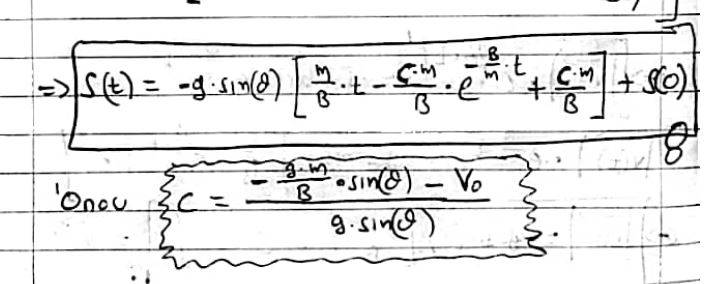C is also pretty big, not to mention t is also in the exponent as well, I will be lost in the calculations...
I can't believe university physics has so many calculations.

So far I've done, Calculus, Vector Calculus, Complex Analysis (Applied maths), differential equations, Laplace/Fourier transformations, Electrical Circuits 1,2,3 but this physics has the most calculations I've ever seen so far and it's just the beginning, We still didn't cover energy, work, circular motion, torque, springs and a lot of other stuff. Plus we are going to learn some Theory of Relativity as well at the end of the semester.

I'll discuss this with my tutor as well.

•Lnewqban
You are making your work unnecessarily complicated and it's not only the use of vectors. You are looking for the maximum distance up the plane, not the time it takes to get there, so skip the time. Your differential equation is $$m\frac{dv}{dt}=-Bv-F$$ where ##F=mg\sin\!\theta.## Now you apply the usual transformation, ##\dfrac{dv}{dt}=\dfrac{dv}{dx}\dfrac{dx}{dt}=v\dfrac{dv}{dx}## to get $$mv\frac{dv}{dx}=-Bv-F~\implies~\frac{v~dv}{Bv+F}=-\frac{1}{m}dx.$$ If you integrate both sides over the appropriate limits of integration, your desired answer will drop out immediately. You can check whether it is correct by doing a series expansion in ##B## around ##B=0##. The leading term should be what you expect when there is no retarding force. It works.

•PeroK, babaliaris, bob012345 and 1 other person
I can't show you the exercise since it is written in Greek.

It would be helpful to post the original problem even if it is in Greek because we may get a fuller sense of it. This problem seems undefined to me. If the ramp is frictionless, the ball will not roll but will only slide. But what happens off the ramp? Is the level ground frictionless or not? If not, the ball will start rolling instead of sliding.

•Lnewqban
I can't show you the exercise since it is written in Greek.

It would be helpful to post the original problem even if it is in Greek because we may get a fuller sense of it. This problem seems undefined to me. If the ramp is frictionless, the ball will not roll but will only slide. But what happens off the ramp? Is the level ground frictionless or not? If not, the ball will start rolling instead of sliding.
If the ramp is frictionless, it makes no difference whether the mass is in the shape of a ball or a block. The solution is the same.

•PeroK
But what happens off the ramp? Is the level ground frictionless or not? If not, the ball will start rolling instead of sliding.
The object starts on the ramp, is going uphill and the velocity becomes zero while on the ramp.. The level ground is not relevant for the problem.

•bob012345
You are making your work unnecessarily complicated and it's not only the use of vectors. You are looking for the maximum distance up the plane, not the time it takes to get there, so skip the time. Your differential equation is $$m\frac{dv}{dt}=-Bv-F$$ where ##F=mg\sin\!\theta.## Now you apply the usual transformation, ##\dfrac{dv}{dt}=\dfrac{dv}{dx}\dfrac{dx}{dt}=v\dfrac{dv}{dx}## to get $$mv\frac{dv}{dx}=-Bv-F~\implies~\frac{v~dv}{Bv+F}=-\frac{1}{m}dx.$$ If you integrate both sides over the appropriate limits of integration, your desired answer will drop out immediately. You can check whether it is correct by doing a series expansion in ##B## around ##B=0##. The leading term should be what you expect when there is no retarding force. It works.
Wow, I think I'm getting it! So you basically create an association between distance and velocity. In other words, you express the distance as a function of velocity and once you figure that out you will just plug in 0 for velocity, yielding the answer of the exercise immediately. Is this correct?

The reason I didn't think like that it's because most of my experience in Physics comes from high school where we were always taking a 2-way step (first find the time and then use it to find something else) or something similar.

Wow, I think I'm getting it! So you basically create an association between distance and velocity. In other words, you express the distance as a function of velocity and once you figure that out you will just plug in 0 for velocity, yielding the answer of the exercise immediately. Is this correct?
Yes, the idea is to find the velocity as a function of position and relate position and velocity at point A (where you know the velocity and the position) to an unknown position where the velocity is zero. It is sometimes called a first integral.
The reason I didn't think like that it's because most of my experience in Physics comes from high school where we were always taking a 2-way step (first find the time and then use it to find something else) or something similar.
If you stop and think about it for a moment, problems that are solved using mechanical energy conservation do just that: relate position and speed at point A with position and speed at point B. So if you are given three of the quantities, you can find the fourth mechanical using energy conservation and bypass the need for finding the time. I am sure you solved such problems in high school using mechanical energy conservation. You probably didn't realize at the time that it simplified the two-step process when you are not specifically asked to find the transit time from A to B.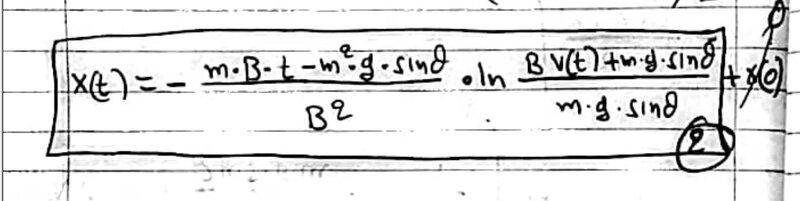I ended up with the above. I still have time inside this equation, so I'm not sure how to find the distance traveled until it stops. I can set ##v(t) = 0## but what is the time at that point? I do not know... I need to take the derivative, find v(t), and set that to zero in order to figure out the time.

Is the above formula correct?

#### Attachments

• solution.pdf
325.7 KB · Views: 43
It is not correct. Think of the transformation this way ##\dfrac{dv(t)}{dt}\rightarrow v(x)\dfrac{dv(x)}{dx}.## Then you separate variables and you have an integral over variable ##v## on the left-hand side and another integral over variable ##x## on the right-hand side.

•babaliaris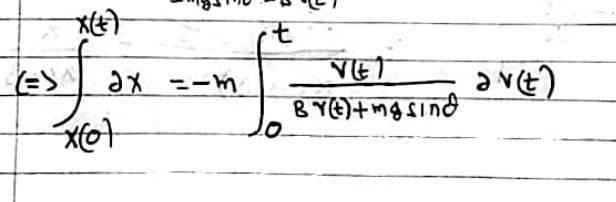But this is exactly what I got. And solving this for x(t) I got the following:View attachment 299297
But this is exactly what I got. And solving this for x(t) I got the following:
View attachment 299298
It may be what you got but it is not correct because there should be no time variable ##t## in it. Start with
$$\frac{v~dv}{Bv+F}=-\frac{1}{m}dx.$$Now integrate both sides:
$$\int\frac{v~dv}{Bv+F}=-\int\frac{1}{m}dx.$$Remember that an integral is a summation. On the left-hand side we are adding pieces that look like ##\dfrac{v~dv}{Bv+F}## where ##v## is the instantaneous velocity at position ##x## and ##dv## is an increment of the velocity that takes us to the next instantaneous velocity. On the right-hand side we are adding pieces that look like ##-\dfrac{1}{m}dx## where ##x## is the instantaneous position when the velocity is ##v## and ##dx## is an increment of the position that takes us to the next instantaneous position. There is no variable ##t## anywhere.

All you have to do is set the limits of integration. In other words, at what pair of velocity and position should you start adding and at what pair of velocity and position should you stop adding? These pairs are the lower and upper limits of integration.

•babaliaris and nasu
LoL I found my mistake: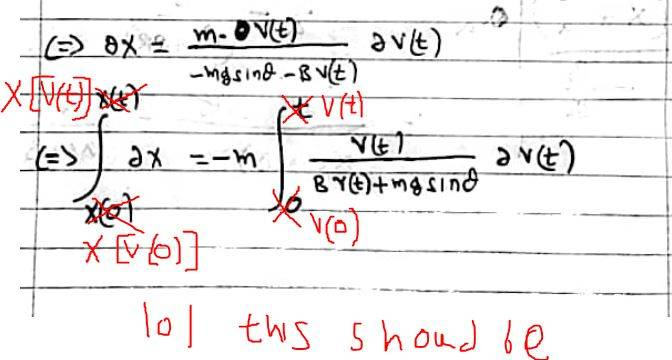That's good. Now you have to do the integrals.

•babaliaris
LoL I found my mistake:
View attachment 299301
I would usually resolve things using a constant of integration, rather than worry about bounds on the integral. E.g. with ##F = 0## we have
$$m\frac{dv}{dt} = -Bv$$$$\frac m v \ dv = -B \ dt$$$$m \ln v = -Bt + C$$Then ##t = 0, v = v_0## gives:
$$C = m \ln v_0$$Hence$$Bt = m \ln v_0 - m\ln v = m \ln \frac {v_0}{v}$$Or, to get ##v(t)##$$m \ln v = -Bt + C$$$$v = Ae^{-\frac{Bt}{m}}$$Where ##A = e^{C/m}##. And, ##t = 0, v = v_0## gives ##A = v_0##, hence
$$v = v_0 e^{-\frac{Bt}{m}}$$

Last edited:
•babaliaris
I would usually resolve things using a constant of integration, rather than worry about bounds on the integral. E.g. with ##F = 0## we have
$$m\frac{dv}{dt} = -Bv$$$$\frac m v \ dv = -B \ dt$$$$m \ln v = -Bt + C$$Then ##t = 0, v = v_0## gives:
$$C = m \ln v_0$$Hence$$Bt = m \ln v_0 - \ln v = m \ln \frac {v_0}{v}$$Or, to get ##v(t)##$$m \ln v = -Bt + C$$$$v = Ae^{-\frac{Bt}{m}}$$Where ##A = e^{C/m}##. And, ##t = 0, v = v_0## gives ##A = v_0##, hence
$$v = v_0 e^{-\frac{Bt}{m}}$$
I believe you are right. It's probably easier this way and then using the initial value you can go and calculate the C constant because the bounds gave me a very hard time solving the integral. Plus when you have to use u-substitution to solve the integral things start getting weird since you have something like
$$\int_{u[s[v(0)]]}^{u[s[v(t)]]} u[s[t]] \cdot du[s(t)]$$

Last edited:
By the way, I need to do some research, since it's the first time doing these kinds of integration and it seems a bit compilating.

Are you guys sure that this is correct?
##\int_{x[v(0)]}^{x[v(t)]}dx(t) = \int_{v(0)}^{v(t)}dv(t)##

The solution in my notes (professor's solution) does something like:
##\int_{v(0)}^{v(x)}dv(t) = \int_{0}^{x}dx(t)##

First time I'm confused about integration bounds since these kinds of thoughts were not something
I had to think about, while I was learning differential equations in math class.

It's the same thing isn't itx(0) = 0, this is why the integral on the right goes from 0 to x(t).

In other words, it doesn't matter which bound I decide to use as the input of the other, since
both of these quantities relate to each other.

So i can have:
0 to x(t) and v(0) to v[x(t)] or
v(0) to v(t) and x[(v(0)] to x[v(t)] where ## x[v(0)] = 0 ##

Last edited:
I would usually resolve things using a constant of integration, rather than worry about bounds on the integral. E.g. with ##F = 0## we have
$$m\frac{dv}{dt} = -Bv$$$$\frac m v \ dv = -B \ dt$$$$m \ln v = -Bt + C$$Then ##t = 0, v = v_0## gives:
$$C = m \ln v_0$$Hence$$Bt = m \ln v_0 - m\ln v = m \ln \frac {v_0}{v}$$Or, to get ##v(t)##$$m \ln v = -Bt + C$$$$v = Ae^{-\frac{Bt}{m}}$$Where ##A = e^{C/m}##. And, ##t = 0, v = v_0## gives ##A = v_0##, hence
$$v = v_0 e^{-\frac{Bt}{m}}$$
The derivation is correct but I don't believe it applies here. How does one find the maximum distance up the incline? According to this expression the object stops when ##t ## is infinite. Surely it comes to instantaneous rest sooner than that because it is on an incline. Also, when you ignore air drag (##B=0##), your expression reduces to ##v=v_0##. The correct expression must reduce to ##v=v_0-gt\sin\!\theta##.

I'd rather worry about the bounds on the integral because the answer drops out immediately.

•babaliaris
My professor also uses bounds instead of solving the Indefinite integral and then calculating the C constant.

I would actually explain things differently. If we start from the simple example:
$$\frac{1}{v} \frac{dv}{dt} = -B$$Then we integrate with respect to ##t##:
$$\int_{0}^{t} \frac 1 v \frac{dv}{dt} \ dt = -\int_{0}^{t} B \ dt$$Now, the LHS can be transformed using the substituton rule and the RHS can be integrated directly:
$$\int_{v(0)}^{v(t)} \frac 1 v dv = -Bt$$And, now the LHS can be integrated to give:
$$\ln[v(t)] - \ln[v(0)] = -Bt$$

•babaliaris
This is from a different exercise but it has the same idea (with the one of this post) in order to get s(t) from the s[v(t)] relation: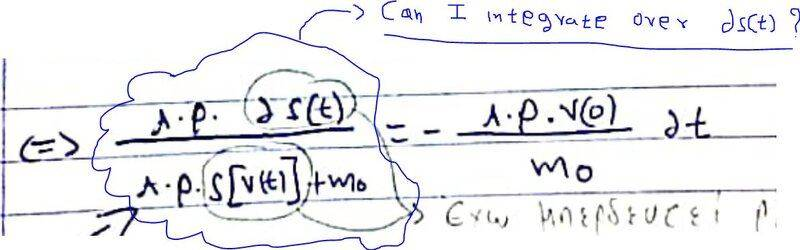I'm not sure if I can integrate over ##ds(t)##. My professor indeed does it but he doesn't show the dependency of each variable so I don't know if s depends on t or v(t).

I would actually explain things differently. If we start from the simple example:
$$\frac{1}{v} \frac{dv}{dt} = -B$$
That is not applicable here. The mass is sliding up an incline at angle ##\theta##. The appropriate equation is $$\frac{dv}{dt} = -Bv-g\sin\!\theta.$$Even if one chooses to find an expression for the velocity as a function of time instead of as a function of position, there should be a ##g\sin\!\theta## somewhere on the right hand side.

Maybe I am not understanding the relevance of ##v=v_0e^{-\frac{B}{m}t}## to OP's original problem. In my method one has a definite integral $$\int_{v_0}^0\frac{v~dv}{Bv+F}=-\frac{1}{m}\int_0^L dx$$where ##F=mg\sin\!\theta.## All one has to do is two integrals and then solve for the distance traveled ##L##.

•babaliaris
Maybe I am not understanding the relevance of ##v=v_0e^{-\frac{B}{m}t}## to OP's original problem.

I definitely did a mistake there (x(t) is not exponential), but you can definitely calculate the velocity as a function of time, and then using ##\frac{dx}{dt}##, you can integrate to find the position function.

That is not applicable here. The mass is sliding up an incline at angle ##\theta##.
It was only an example to show how to use the constant of integration.

•babaliaris
It was only an example to show how to use the constant of integration.
Thanks for the clarification. I think we've been down that road before so I will leave it at that.

•PeroK